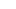# Frog Math Worksheets (2nd-4th grade)

Kids will have fun practicing fractions, multiplication, counting, tally marks, and more with these Frog Math Worksheets.  This super cute Frog Math is for 2nd grade, 3rd grade, and 4th grade students. Use this math frog during the spring as a fun April or May free math worksheets. Simply download pdf file with math fun worksheets and you are ready to spin and learn while having FUN!## Frog math

This Frog Math Worksheets  pack is aimed at children in grade 2, grade 3 and grade 4; it contains over 30 pages for fun, spinning activities to help children become more confident with basic math concepts. These math fun worksheets are perfect for spring math that keeps kids engaged. Whether you are a parent, teacher, or homeschooler – these happy frog math activities are a great way to sneak in a variety of math practice with middle elementary age students.

## Frog math worksheets

Start by scrolling to the bottom of the post, under the terms of use, and click on the text link that says >> Download <<. The pdf file will open in a new window for you to save the freebie and print the template.## Math frog

This 34-page Frog-Themed Spinner math Fun printable packs includes are variety of activities:

• Spin and Shade the Abacus – Spin a number and shade the correct beads on the abacus.
• Spin and Graph Numbers – Spin a number and graph your answers.
• Spin and Add – There are three different pages, spin and add the number. Adding the numbers 1 through 10## Math fun worksheets

• Spin and Subtract – There are three different pages, spin and subtract the number. Subtracting the numbers 1 through 10
• Spin and Multiply – There are three different pages, spin and multiply the number. Multiplying the numbers 1 through 10
• Spin and Divide – There are three different pages, spin and divide by the number. Divide by the numbers 2, 3, 4, 5, 6 and 10## Spring Math

• Spin and Shade Multiplication – There are three different pages, multiplying by itself, 5’s and 10’s
• Spin Greater Than/Less Than/ Equal to – There are two different pages. Spin a number, write it down and add the correct sign
• Spin There are two different pages. Spin a number and add the correct time.• Spin and Match the Tally Marks – There are two different pages. Spin a tally mark and shade in the correct matching number.
• Spin and Match the Roman Numerals – There are two different pages. Spin a Roman Numeral  and shade in the correct matching number.
• Spin and Graph Fractions – There are three different pages. Spin a fraction and graph your spins.
• Spin and Write Equivalent Fractions – There are three different pages. Spin a fraction, write it down and write two more equivalent fractions.## Frog Printables

Looking for more frog worksheets and frog games for kids? Check out these free resources for kids of all ages:

## Spring Science

Check out these outrageously FUN spring science experiments for kids:

• This set is for personal and classroom use only.
• This printable set may not be sold, hosted, reproduced, or stored on any other website or electronic retrieval system.
• Graphics Purchased and used with permission